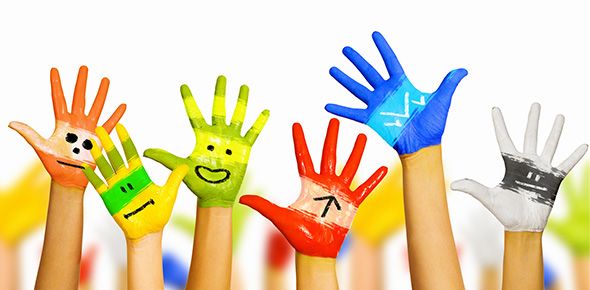# 信王府入编八卦能力测验（乙卷）

30 | Total Attempts: 364Settings• 1.
信王殿下名讳是以下哪一个？
• A.

李枣儿

• B.

崇祯

• C.

朱由检

• D.

朱由枨

• E.

朱由校

• 2.
崇祯生辰月日是以下哪一个？
• A.

三月十九

• B.

腊月二十四

• C.

腊月二十五

• D.

八月二十三

• E.

二月二十五

• 3.
崇祯常常弹的那张琴名为？
• A.

大圣遗音

• B.

崩霆

• C.

绿绮

• D.

翔凤

• E.

不系舟

• 4.
在书法学习方面，据载，崇祯临习过的书法家作品不包括以下哪位的？
• A.

欧阳询

• B.

虞世南

• C.

赵孟頫

• D.

董其昌

• E.

王羲之

• 5.
崇祯冬天若有平台召对，所着貂裘最可能是以下哪一种？
• A.

紫貂

• B.

雪貂

• C.

金貂

• D.

银貂

• E.

花貂

• 6.
根据现存史料实物，以下哪一句最不可能为崇祯所御书过？
• A.

上将威严细柳营

• B.

主宰一气生群生

• C.

桃花马上请长缨

• D.

花发南枝新栋宇

• E.

山月照弹琴

• 7.
天启崇祯兄弟从小感情甚笃。崇祯年间，使得皇帝睹物思人的那件天启亲手所作的东西是
• A.

旋转木马

• B.

旧水戏轮轴

• C.

正阳琴

• D.

檀木奉圣夫人像

• E.

沉香木假山

• 8.
对于比自己年长22岁的杨嗣昌，崇祯有很深的感情。这段感情的起源是
• A.

《地官集》

• B.

天启间杨鹤父子被排挤病辞

• C.

崇祯四年皇帝改判杀杨鹤为戍

• D.

《武陵竞渡略》

• E.

崇祯御赐墨宝

• 9.
夏日着珍珠暑衫在寝宫梳妆，被进门来看见此景的崇祯忍不住亲昵调戏，于是反给他一巴掌的是
• A.

范选侍

• B.

田皇贵妃

• C.

周皇后

• D.

袁皇贵妃

• E.

刘妃

• 10.
崇祯最喜欢的茶是
• A.

涌溪火青

• B.

西湖龙井

• C.

六安瓜片

• D.

都匀毛尖

• E.

龙凤团茶

• 11.
如果面前有数碗吃食，崇祯根据自己口味会选择哪碗？
• A.

鹌鹑馉饳

• B.

燕窝

• C.

鱼翅

• D.

水晶鲈鱼烩

• E.

桂花杏酪

• 12.
崇祯喜欢的花有以下哪种？
• A.

鸡冠花

• B.

• C.

牡丹

• D.

莲花

• E.

黄梅

• 13.
崇祯正式被上的谥（庙）号不包括以下哪个？
• A.

思宗烈皇帝

• B.

绍天绎道刚明恪俭揆文奋武敦仁懋孝烈皇帝

• C.

威宗庄皇帝

• D.

毅宗烈皇帝

• E.

庄烈愍皇帝

• 14.
下列哪个是崇祯殊为喜爱的乐器之一？
• A.

• B.

• C.

琵琶

• D.

• E.

• 15.
崇祯驾崩之后，明亡。下列各项，哪个是后世为了纪念他，所托的神明偶像之一？
• A.

摩利支天

• B.

太乙雷声应化天尊王善王灵官

• C.

沉思罗汉

• D.

朱天菩萨光明佛

• E.

婆竭龙王

• 16.
崇祯登基的地点是
• A.

中极殿

• B.

皇极殿

• C.

乾清宫

• D.

文华殿

• E.

武英殿

• 17.
受罚宫女夜里提铃唱“天下太平”，声音哀戚，为崇祯所闻，于是被唤去问名。崇祯怜悯其苦，遂免责罚。宫女名字是
• A.

叶子眉

• B.

阿奇

• C.

曹静照

• D.

韩翠娥

• E.

褚贞娥

• 18.
崇祯年间，京师里内外勋戚众多，其中以谁家最为侈荡出名，为各大户所诟病？
• A.

嘉定伯家

• B.

太康伯家

• C.

田戚畹家

• D.

万驸马家

• E.

袁皇亲家

• 19.
以下那句话是崇祯对天启所说？
• A.

此便是召对了么？

• B.

军心未一，不可以战。

• C.

亦一时精神所寄也。

• D.

这个官儿我可做得否？

• E.

有人欺负我。

• 20.
丙戌年中，有一道圣旨斥责私自出击的将领，说：“奉天承运，皇帝诏 曰：咱老子叫你不要往汉中去，你强要往汉中去，如今果然折了许多兵马。驴毬子入你妈妈的屄！钦此！”这道圣旨出自
• A.

李闯王

• B.

崇祯

• C.

皇太极

• D.

八大王

• E.

天启

• 21.
崇祯元年，奉旨书写乾清宫匾额“敬天法祖”的人是
• A.

高时明

• B.

高起潜

• C.

刘若愚

• D.

王之心

• E.

曹化淳

• 22.
信王出邸前，所居的潜邸是
• A.

钟粹宫

• B.

长春宫

• C.

玉熙宫

• D.

勖勤宫

• E.

慈宁宫

• 23.
以下哪位不是崇祯的皇子？
• A.

朱慈烺

• B.

朱慈焕

• C.

朱慈炅

• D.

朱慈灿

• E.

朱慈炡

• 24.
原文：文武官品服色，祖制既定，奉行已久；惟是武弁概服獅子。上至是，重行申飭：『武弁三四品，俱炤制服虎豹』。至內奄從無定式，蓋直擯之洒掃服役之末，祖制良有深意：雖太監極品，止於正四品，間有賜蟒玉者，亦後來之濫觴，非祖制也。问题：以何依据更易服制？
• A.

《山海经》

• B.

《南山经》

• C.

《朱子家礼》

• D.

《尚书》

• E.

《仪礼》

• 25.
某人记载，其一日过长安书肆，看见《皇明泳化编》一部，想买，书商故意抬价，他便说：「那就再等等。」及旋骑再访，则说，已经卖掉了。他问书商，书商说买者乃囗囗囗囗囗也。他很惊讶。数年后想起此事感慨，说：“（买书人）后死闯贼难，方知观人必於其微。”故事中，买书人是谁？
• A.

黄道周

• B.

巩永固

• C.

夏允彝

• D.

王世德

• E.

李国祯